This is a Clilstore unit. You can .

# Solids-3D

List of Geometrics Shapes - 3D Shapes

 Cube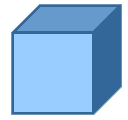Cubes have 6 faces, 12 edges and 8 vertices. All sides on a cube are equal length. All faces are square in shape. A cube is a type of cuboid. Cuboid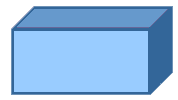Cuboids have 6 faces, 12 edges and 8 vertices. All the faces on a cuboid are rectangular. SphereSpheres have either 0 or 1 faces, 0 edges and 0 vertices. EllipsoidEllipsoids have either 0 or 1 faces, 0 edges and 0 vertices. Cylinder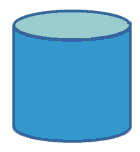Cylinders have either 2 or 3 faces, 0 or 2 edges, and 0 vertices. Cone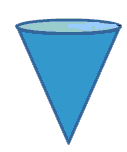Cones have either 1 or 2 faces, 0 or 1 edges, and 1 apex (which is described by some mathematicians as a vertex). Triangular Prism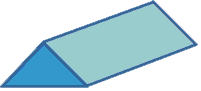Triangular Prisms have 5 faces, 9 edges, and 6 vertices. The two faces at either end are triangles, and the rest of the faces are rectangular. Hexagonal PrismHexagonal Prisms have 8 faces, 18 edges, and 12 vertices. The two faces at either end are hexagons, and the rest of the faces are rectangular. Triangular-based Pyramid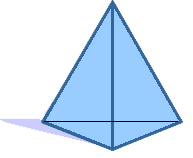Triangular-based pyramids have 4 faces, 6 edges and 4 vertices. The base is a triangle. All of the faces are triangular. If the triangular faces making up the prism are all equilateral, then the shape is also called a Tetrahedron. Square-based Pyramid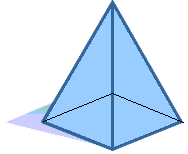Square based pyramids have 5 faces, 8 edges and 5 vertices The base is a square. All the other faces are triangular. Hexagonal Pyramid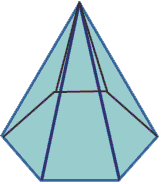Hexagonal pyramids have 7 faces, 12 edges, and 7 vertices. The base is a hexagon. All of the other faces are triangular.

The 5 Platonic Solids

The platonic solids form a set of 5 polyhedra with the following special properties:

• the faces of the platonic solids have to be regular and congruent.
• the same number of faces meet at each vertex.
 TetrahedronA Tetrahedrons is the same as a triangular pyramid. They have 4 triangular faces, 6 edges and 4 vertices. A regular tetrahedron has equilateral triangles for its faces, and is one of the 5 platonic solids. Cube (regular hexahedron)Cubes have 6 faces, 12 edges and 8 vertices. All sides on a cube are equal length. All faces are square in shape. A cube is a type of cuboid and is one of the 5 platonic solids. OctahedronOctahedrons are a shape with 8 faces, 12 edges and 6 vertices. A regular octahedron has equilateral triangles for its faces, and is one of the 5 platonic solids. DodecahedronDodecahedrons are a shape with 12 faces, 30 edges and 20 vertices. A regular dodecahedron has regular pentagons for its faces, and is one of the 5 platonic solids. IcosahedronIcosahedron are a shape with 20 faces, 30 edges and 12 vertices. All the faces are triangles. A regular icosahedron is one of the 5 platonic solids with all faces being equilateral triangles.

Short url:   https://clilstore.eu/cs/5500